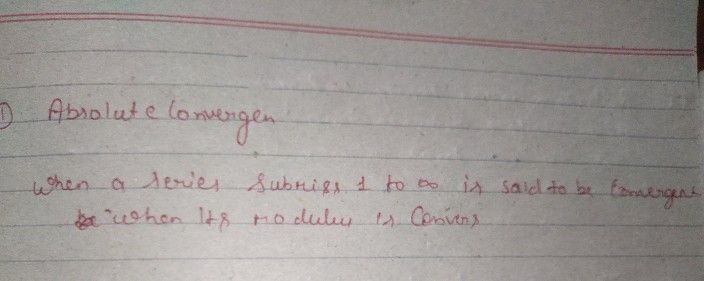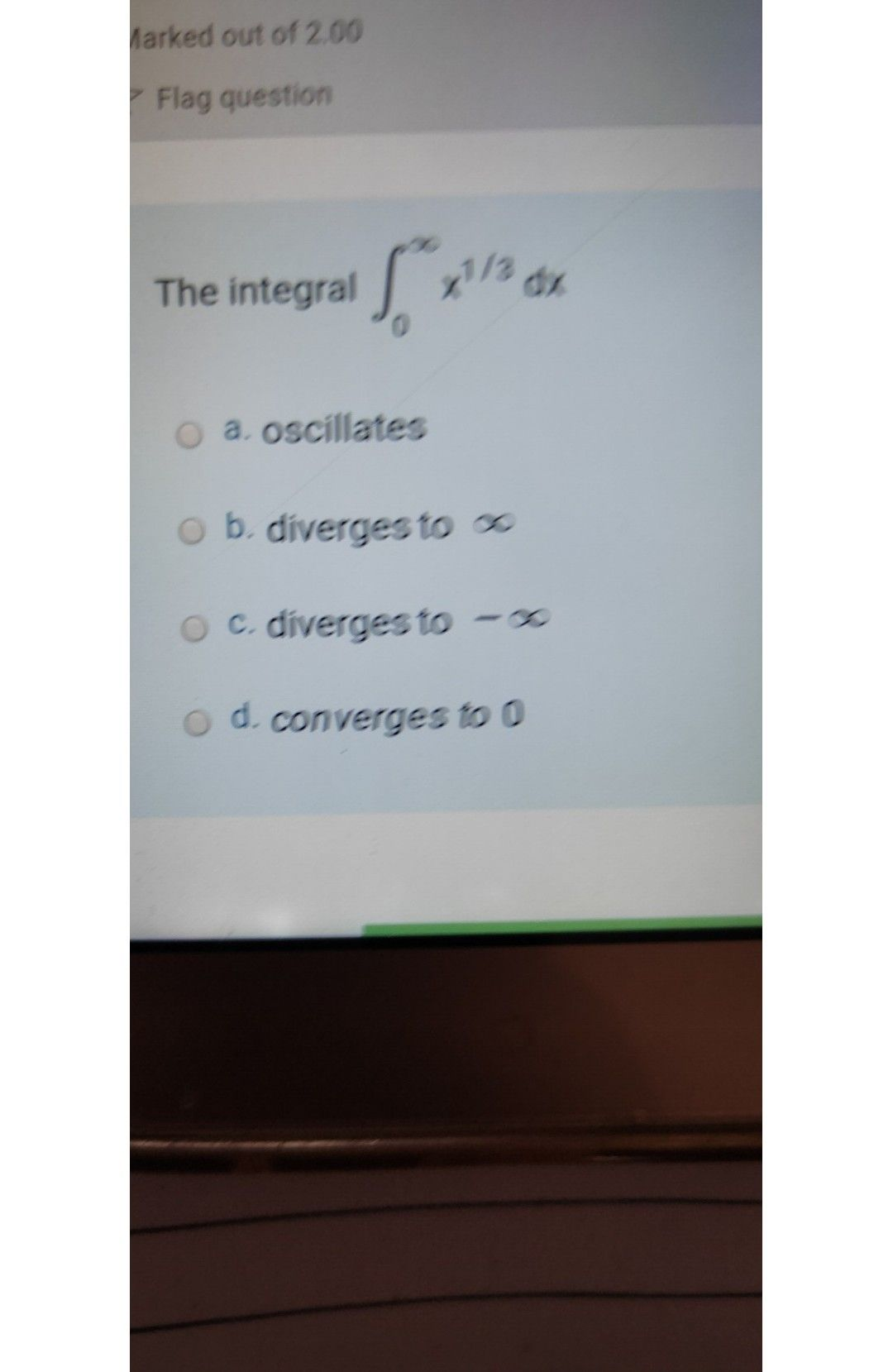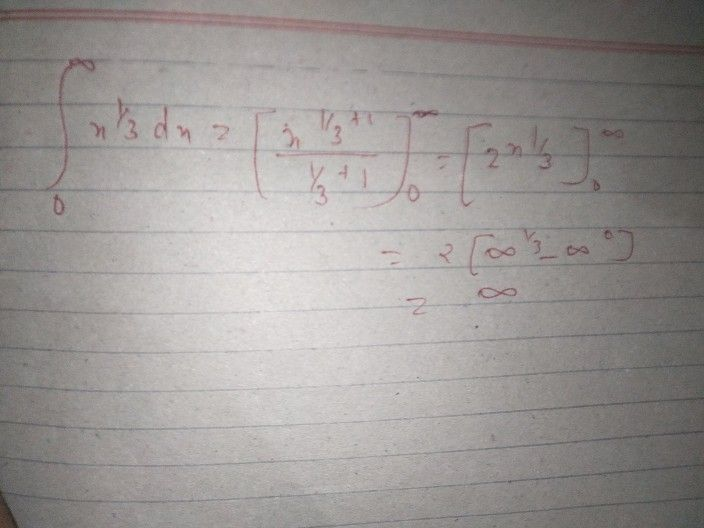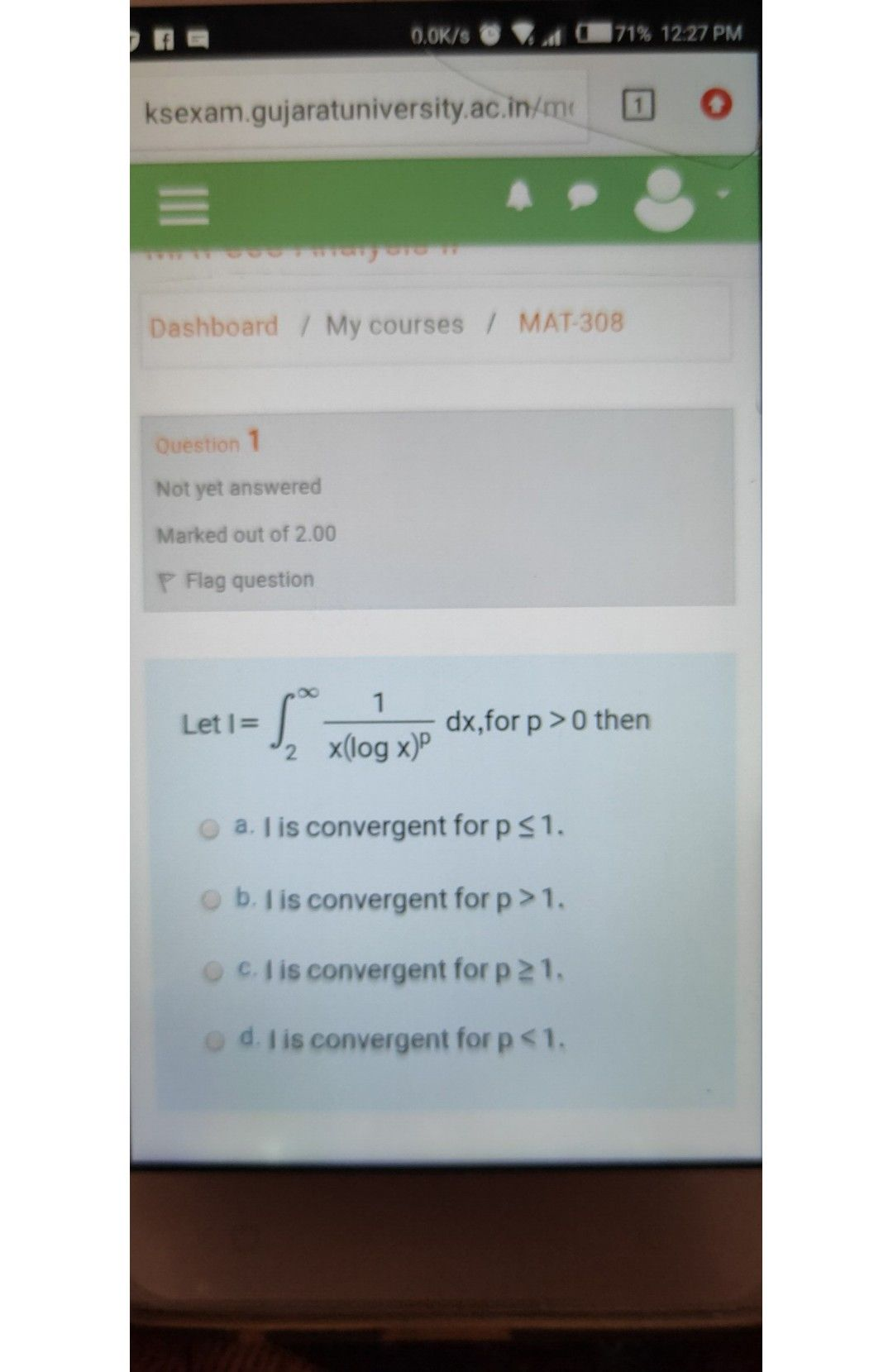Symbol
ProblemMultiple Choice Type Questions : Select (a), (b), (c) ur tun 1. An absolutely convergent series out of the following series is: +.... (b) $1-\dfrac {1} {2}+\dfrac {1} {3}+\dfrac {1} {4}+$ (a) $1-\dfrac {x^{2}} {21}+\dfrac {x^{4}} {41}-\dfrac {x^{6}} {61}+$ $\left(4\right)$ $\sum \dfrac {\left(-1\right)^{n+1}} {\sqrt{n} }$ $\left(kangax$ $\left(c\right)$ $\sum \dfrac {\left(-1\right)^{n+1}} {3n-2}$ 2. Which of the $\left(ollowing$ is a convergent series ? (a) nE =1 $\left(-1\right)^{n-1}\dfrac {1} {n}$ (b) $\sum _{n=1}a_{n},whc\pi c$ $a_{2}=2$ V ncN 3". (Kanpur, 2 (c) nE =1 n 3/4 (d) 3. The $scios1-\dfrac {1} {3}+\dfrac {1} {3^{2}}-\dfrac {1} {3^{3}}+$ Divergent (b) Conditionally convergent (a) ()Absolutely $c0nycrgcn$ (d) None of these. 4. The $scnics1-\dfrac {1} {\sqrt{2} }+\dfrac {1} {\sqrt{3} }-\dfrac {1} {\sqrt{4} }+$ (a) $Diycrgcn$ (b) Conditionally convergent (c) Absolutely convergent (d) None of these. 5. The series $1-\dfrac {1} {2^{2}}+\dfrac {1} {3^{2}}-\dfrac {1} {4^{2}}+$ ..... is: (a) Oscillatory (b) Divergent (c) Abeolutely convergent (d)Conditionally convergent. 6. Wh.ch one of the following does not give absolute convergence of the seric (a) Root test (b) Comparison test (c) Ratio test (d) Leibnitz's 7. TL.A.S. (Prel.). Consider the following statements : converges. Of these statem 1. <u converges $2.\sum y_{n}$ converges $3.\sum |x_{n}|con$ (a) $1impllcs2$ (b) 3 implies I (c) 1 implies 3 (d) 2 implies 8. If E u, is a series of positive terms, then: ILA.S. (Prel.k (a) Convergence of $\sum \left(-1\right)^{n}$ u, convergence of 2 u, (b) Convergence of Z u, convergence of E(- 1)" () Convergence of Z (- 1)" u, divergence of E u,
Other
Search count: 106
SolutionQanda teacher - ShefaliStudentQanda teacher - ShefaliIf you have any doubts please do let me know dear. if you are satisfied with my answer please do LIKE and give a 5 STAR RATING and coins as THANK U GIFT..! ALL THE BEST..!!! Please ask through preferred match (from my profile) if you have more questions.StudentQanda teacher - Shefali
sorry s parmar I can solve one question one timer according to qanda prospective uh can again send it on prefferdStudent
please its my test